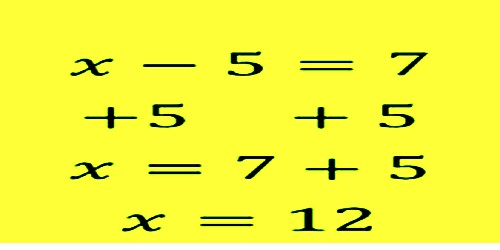Mathematic

# Subtraction EquationsSubtraction equations

To solve an equation means to find a value for the variable that makes the equation true. Whatever you do to one side of the equation, you must also do to the other side. An equation is a mathematical statement such that the expression on the left side of the equals sign (=) has the same value as the expression on the right side. An example of an equation is 6 – 4 = 2.

One of the terms in an equation may not be know and needs to be determined. Often this unknown term is represented by a letter such as x. (e.g. x – 4 = 2).

The solution of an equation is finding the value of the unknown x. To find the value of x we can use the additive equation property which says: The two sides of an equation remain equal if the same number is added to each side.

Example:

x – 5 = 7

x – 5 + 5 = 7 + 5

x – 0 = 12

x = 12

Check the answer by substituting the value of x (12) back into the equation.

12 – 5 = 7

Explanation:

Subtraction equations – 3 digit

An equation is a mathematical statement such that the expression on the left side of the equals sign (=) has the same value as the expression on the right side. An example of an equation is 600 – 400 = 200.

One of the terms in an equation may not be know and needs to be determined. Often this unknown term is represented by a letter such as x. (e.g. x – 400 = 200).

The solution of an equation is finding the value of the unknown x. To find the value of x we can use the additive equation property which says: The two sides of an equation remain equal if the same number is added to each side.

Example:

x – 500 = 700

x – 500 + 500 = 700 + 500

x – 0 = 1200

x = 1200

Check the answer by substituting the value of x (1200) back into the equation.

1200 – 500 = 700

Subtraction equations – 6 digit

An equation is a mathematical statement such that the expression on the left side of the equals sign (=) has the same value as the expression on the right side. An example of an equation is 600000 – 400000 = 200000.

One of the terms in an equation may not be know and needs to be determined. Often this unknown term is represented by a letter such as x. (e.g. x – 400000 = 200000).

The solution of an equation is finding the value of the unknown x. To find the value of x we can use the additive equation property which says: The two sides of an equation remain equal if the same number is added to each side

Example:

x – 500000 = 700000

x – 500000 + 500000 = 700000 + 500000

x – 0 = 1200000

x = 1200000

Check the answer by substituting the value of x (1200000) back into the equation.

1200000 – 500000 = 700000

Information Source: